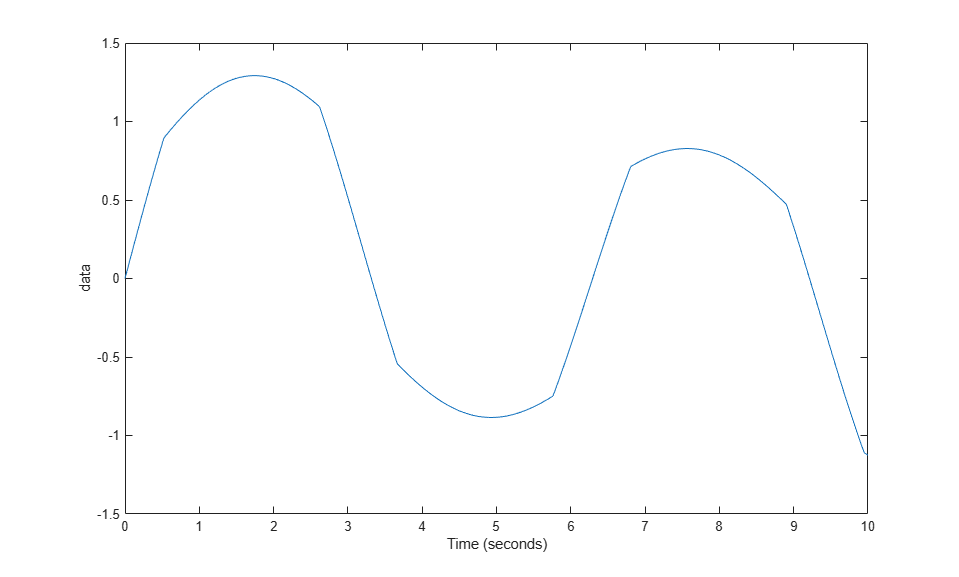# Control Variant Choices in Masked Variant Assembly Subsystem Block Using Mask Parameter Object

This example shows how to specify variant choices and set an active choice in a masked Variant Assembly Subsystem block using a mask parameter object.

### Explore Model

Open the model `slexVariantAssemblySubsystemWithMask`, which contains a masked Variant Assembly Subsystem block, `Controller`.

```open_system('slexVariantAssemblySubsystemWithMask') ```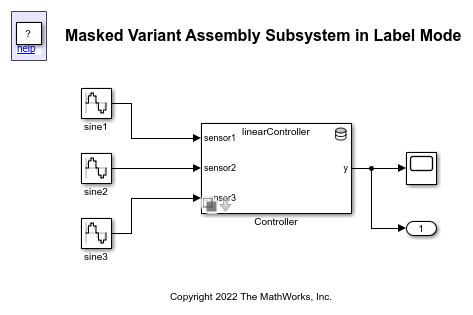### Define Classes for Reference Models

In this example, the `vas_controller` class is a superclass that includes a property and two methods. The `getVariantChoices` method returns a cell array of variant choices to be added to the Variant Assembly Subsystem block. From the list of choices, the `setActiveVariant` method sets the choice, specified as `subsystemFile`, to be active.

```type vas_controller.m ```
```classdef (Abstract) vas_controller properties subsystemFile char end methods (Static) function list = getVariantChoices() list = {'linearController','nonlinearController'}; end end methods function setActiveVariant(obj,blk) set_param(blk,'LabelModeActiveChoice',obj.subsystemFile) end end end ```

The two classes `vas_linearController` and `vas_nonlinearController` inherit from the `vas_controller` superclass. The `vas_linearController` class stores the name of the active choice as `linearController` in the inherited property `subsystemFile`. The class also stores numerator and denominator values used in the Discrete Transfer Fcn block of the linearController model.

```type vas_linearController.m ```
```classdef vas_linearController < vas_controller properties numerator denominator end methods function obj = vas_linearController() obj.numerator = [1 .7]; obj.denominator = [1 .09 0.5]; obj.subsystemFile = 'linearController'; end end end ```

Similarly, the `vas_nonlinearController` class stores the name of the active choice as `nonlinearController` in `subsystemFile`. The class also stores breakpoint and table values used in the 1-D Lookup Table of the nonLinearController model.

```type vas_nonlinearController.m ```
```classdef vas_nonlinearController < vas_controller properties breakpoints table end methods function obj = vas_nonlinearController() obj.breakpoints = -5:5; obj.table = tanh(-5:5); obj.subsystemFile = 'nonlinearController'; end end end ```

### Set Parameter Object Value in Parent Model

Double-click the `Controller` block to open the mask dialog box.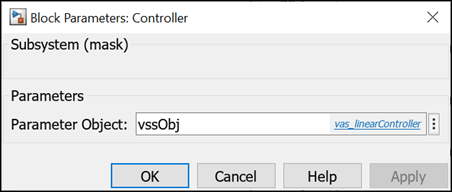The value that you specify for `vssObj` in the mask dialog box is mapped to the mask parameter object `obj`.

Using `obj`, you can instantiate the class `vas_linearController` or `vas_nonlinearController`. You can then call its methods, `getVariantChoices` and `setActiveVariant`.

For example, to specify the variant choices of the Controller block, the Variant choices specifier parameter in the Reference tab of the Block Parameters dialog box is set to `obj.getVariantChoices()`.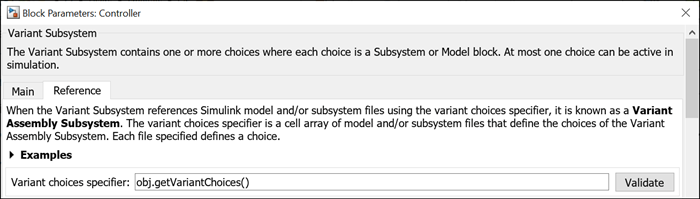To set an active choice in the Variant Assembly Subsystem block, the `obj.setActiveVariant()` method is used in its mask initialization code.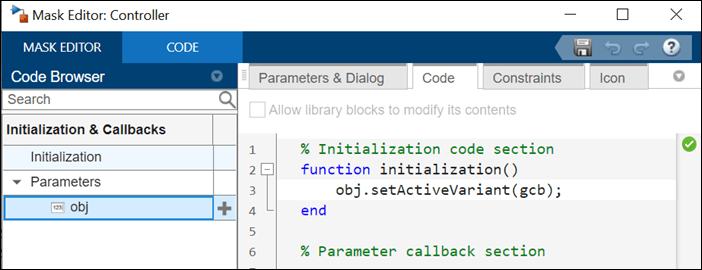### Set Active Choice by Instantiating Classes Using Mask Parameter Object

Case 1: linearController Is Active and nonlinearController Is Inactive

When you specify `vssObj` as `vas_linearController`, the value `vas_linearController` is mapped to the mask parameter object `obj`. The Variant choices specifier parameter value `obj.getVariantChoices()` evaluates to a cell array that contains the filenames `linearController` and `nonlinearController` to be added as variant choices to the Controller block. `obj.setActiveVariant(gcb)` in the mask initialization code sets the `linearController` subsystem to be active.

```vssObj = vas_linearController; out = sim('slexVariantAssemblySubsystemWithMask'); plot(out.tout, out.yout); xlabel('Time (seconds)') ylabel('data') ```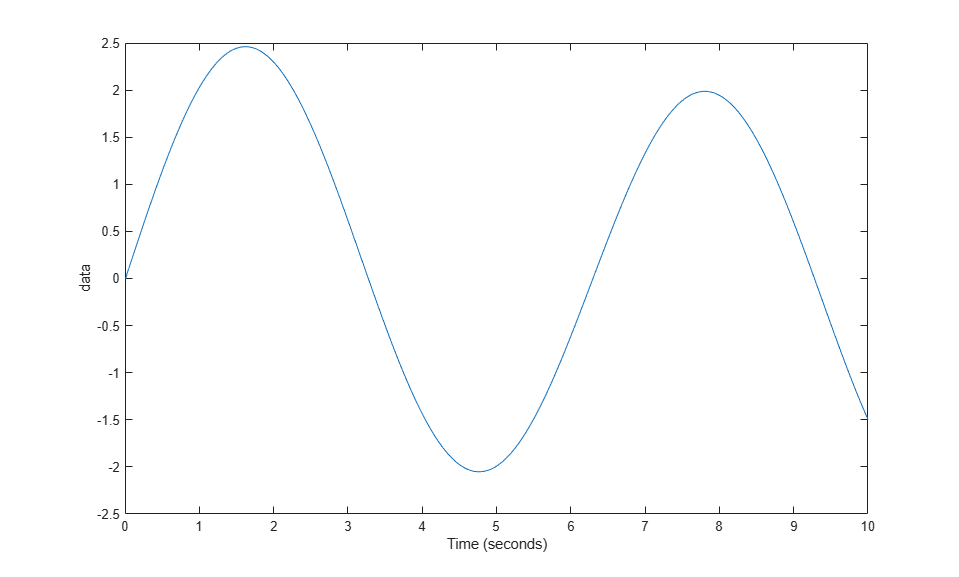Case 2: linearController Is Inactive and nonlinearController Is Active

When you specify `vssObj` as `vas_nonlinearController`, the value `vas_nonlinearController` is mapped to the mask parameter object `obj`. The Variant choices specifier parameter value `obj.getVariantChoices()` evaluates to a cell array that contains the filenames `linearController` and `nonlinearController` to be added as variant choices to the Controller block. `obj.setActiveVariant(gcb)` in the mask initialization code sets the `nonlinearController` subsystem to be active.

```vssObj = vas_nonlinearController; out = sim('slexVariantAssemblySubsystemWithMask'); plot(out.tout, out.yout); xlabel('Time (seconds)') ylabel('data') ```